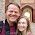## Wednesday, May 1, 2013

### A Command for Randomly Creating Sets of Elements from a Vector

# This command pairs (of arbitrary length) together a list of items randomly.
# I think it is useful for simulations matching individuals together.

pairup = function(x, ncol=2, unmatched.self=T) {

# Calculate the length of the x vector.
xleng = length(x)

# This checks if the input vector is a scalar.
# If it is then it assumes that the number of that scalar is to be the last number is the seqence 1:x
if (xleng==1) x = 1:(xleng=x)
# This command is pretty weird.  I combined two commands to make a single line command.
# It sets the xleng equal to x and x equal to the sequence 1:x

# Calculate the length of each column that will be created.
hleng = floor(xleng/ncol)

# Randomize x
x = x[order(runif(xleng))]

pair = x[1:hleng]
for (i in 2:ncol) pair = cbind(pair,x[((i-1)*hleng+1):((i)*hleng)])

# If there is a odd number of xs then this will match the remaining unmatched x with itself if unmatched.self is T.
if ((unmatched.self)&(floor(xleng/ncol)!=xleng/ncol)) pair=rbind(pair, c(x[ncol*hleng+1]))
if ((!unmatched.self)&(floor(xleng/ncol)!=xleng/ncol)) print(x[!(x %in% pair)])

return (pair)
}

pairup(1:10)
pairup(99)
pairup(letters)
pairup(letters, ncol=3)
pairup(letters, ncol=3, unmatched.self=F)

1.Nice! A 'sample'-based approach I pasted to gist:

https://gist.github.com/anonymous/5504611

1.Looks good! Actually I think your command works better than mine :)

pairup(1:11, ncol=3)
pairup2(1:11, ncol=3)

My command drops one of the values which should be paired with itself. Thanks for posting this code. I really appreciate it.

Francis